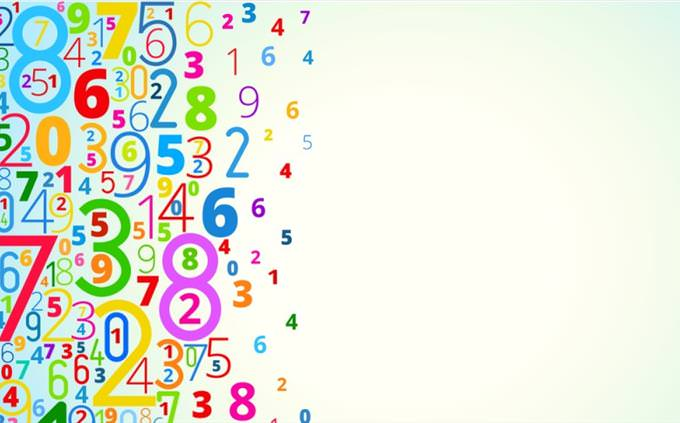# 15 Easy to Challenging Algebra Problems

Are you ready to challenge your brains again in a fun math quiz? Welcome to our Algebra test! You will be given 15 questions with some algebra problems on them. You'll also have 15 minutes to complete the test. Most of these aren't hard, but we did put some tricky ones in there, so consider carefully. Also, obviously, you are not allowed calculators... So have fun and good luck!25 ÷ 5 (10 ? 5) = ?
25
5
1
151 + 1 × ¼ + 1 ? 1 = ?
1.25
1
0.25
27 + 1 x 1 + 8 ÷ 2 = ?
12
5
7
85 + 3 (5 + 3) = ?
29
8
2
648 + 8 ÷ 8 + 8 x 8 – 8 = ?
65
64
16
744 + 1 × 4 + 4 ? 1 = ?
11
16
4
15 + 1 x 0 + 8 ÷ 2 = ?
9
7
2
420 – 20 ÷ ¼ + 1 = ?
-59
-40
40
598 ÷ 4 (4 – 2) = ?
1
6
8
1020 – 4 ÷ ¼ + 8 = ?
12
16
10
620 ÷ 4 x 10 ÷ 2 = ?
25
50
12
13.56 ÷ 2 (2 + 1) = ?
9
3
1
128 ÷ 2 + 4 (8 ? 4) = ?
20
7
4
158 ÷ 4 (4 – 2) = ?
4
8
16
206 – 1 x 0 + 3 ÷ 3 = ?
7
21
18
19